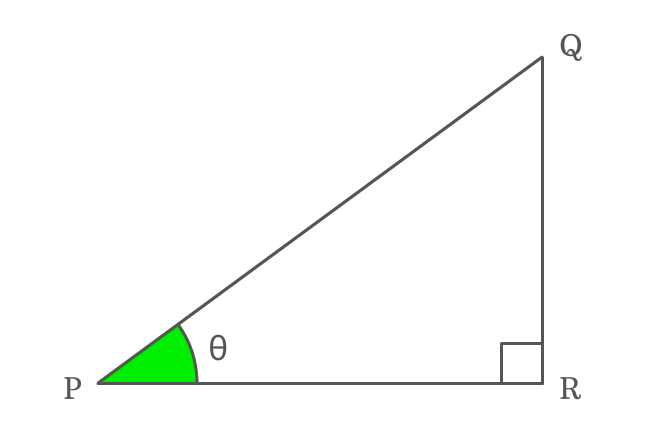# Reciprocal identity of Cosecant function

## Formula

$\sin{\theta} \,=\, \dfrac{1}{\csc{\theta}}$

### Proof

Cosecant is a ratio of lengths of hypotenuse to opposite side and the sine is a ratio of lengths of opposite side to hypotenuse. The cosecant and sine functions are reciprocals mutually. So, the reciprocal of cosecant of angle is equals to sin of angle.

$\Delta QPR$ is a right triangle and the angle of this triangle is theta.#### Write cosecant in its ratio form

Express the cosecant of angle theta ($\csc{\theta}$ or $\operatorname{cosec}{\theta}$) in its ratio form firstly.

$\csc{\theta} \,=\, \dfrac{PQ}{QR}$

#### Write sine in its ratio form

In the same way, express sin of angle theta ($\sin{\theta}$) in its ratio form.

$\sin{\theta} \,=\, \dfrac{QR}{PQ}$

#### Relation between cosecant and sine

Write the value of sin function in its ratio form in reciprocal form and it is useful to prove the relation between cosecant and sine functions in trigonometry.

$\implies \sin{\theta} \,=\, \dfrac{1}{\dfrac{PQ}{QR}}$

$\,\,\, \therefore \,\,\,\,\,\,$ $\sin{\theta} \,=\, \dfrac{1}{\csc{\theta}}$

Therefore, it is proved that the reciprocal of cosecant function is equal to sine function and it will be used as a formula in trigonometric mathematics.

###### Note

The angle of right triangle can be denoted by any symbol but the reciprocal identity of cosecant function must be written in the respective angle.

For example, if $x$ is used to represent angle of right triangle, then

$\sin{x} \,=\, \dfrac{1}{\csc{x}}$

Similarly, if $A$ is used to denote angle of right triangle, then

$\sin{A} \,=\, \dfrac{1}{\csc{A}}$

The reciprocal identity of cosecant function is written in this form everywhere but the only changing factor is angle of the right triangle.

Latest Math Topics
Jun 26, 2023
Jun 23, 2023

Latest Math Problems
Jul 01, 2023
Jun 25, 2023
###### Math Questions

The math problems with solutions to learn how to solve a problem.

Learn solutions

Practice now

###### Math Videos

The math videos tutorials with visual graphics to learn every concept.

Watch now

###### Subscribe us

Get the latest math updates from the Math Doubts by subscribing us.